# Control Flow Testing

It was from the primeval wellspring of an antediluvian passion that my story arises which, like the round earth flattened on a map, is but a linear projection of an otherwise periphrastic and polyphiloprogenitive, non-planar, non-didactic, self-inverting construction whose obscurantist geotropic liminality is beyond reasonable doubt.

— Milinda Banerjee

## Introduction

Control flow testing is one of two white box testing techniques. This testing approach identifies the execution paths through a module of program code and then creates and executes test cases to cover those paths. The second technique, discussed in the next chapter, focuses on data flow.

 Key Point Path: A sequence of statement execution that begins at an entry and ends at an exit.

Unfortunately, in any reasonably interesting module, attempting exhaustive testing of all control flow paths has a number of significant drawbacks.

• The number of paths could be huge and thus untestable within a reasonable amount of time. Every decision doubles the number of paths and every loop multiplies the paths by the number of iterations through the loop. For example:

``` for (i=1; i<=1000; i++)
for (j=1; j<=1000; j++)
for (k=1; k<=1000; k++)
doSomethingWith(i,j,k);
```

executes doSomethingWith() one billion times (1000 x 1000 x 1000). Each unique path deserves to be tested.

• Paths called for in the specification may simply be missing from the module. Any testing approach based on implemented paths will never find paths that were not implemented.

``` if (a>0) doIsGreater();
if (a==0) dolsEqual();
// missing statement - if (a<0) dolsLess();
```
• Defects may exist in processing statements within the module even through the control flow itself is correct.

``` // actual (but incorrect) code
a=a+1;
// correct code
a=a-1;
```
• The module may execute correctly for almost all data values but fail for a few.

``` int blech (int a, int b) {
return a/b;
}
```

fails if b has the value 0 but executes correctly if b is not 0.

Even though control flow testing has a number of drawbacks, it is still a vital tool in the tester's toolbox.

## Technique

### Control Flow Graphs

Control flow graphs are the foundation of control flow testing. These graphs document the module's control structure. Modules of code are converted to graphs, the paths through the graphs are analyzed, and test cases are created from that analysis. Control flow graphs consist of a number of elements:

 Key Point Control flow graphs are the foundation of control flow testing.

Process Blocks

A process block is a sequence of program statements that execute sequentially from beginning to end. No entry into the block is permitted except at the beginning. No exit from the block is permitted except at the end. Once the block is initiated, every statement within it will be executed sequentially. Process blocks are represented in control flow graphs by a bubble with one entry and one exit.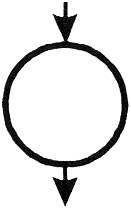Decision Point

A decision point is a point in the module at which the control flow can change. Most decision points are binary and are implemented by if-then-else statements. Multi-way decision points are implemented by case statements. They are represented by a bubble with one entry and multiple exits.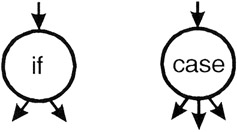Junction Point

A junction point is a point at which control flows join together.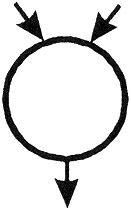The following code example is represented by its associated flow graph: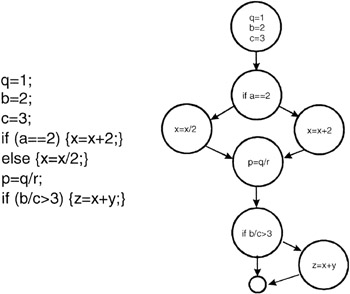Figure 10-1: Flow graph equivalent of program code.

### Levels of Coverage

In control flow testing, different levels of test coverage are defined. By "coverage" we mean the percentage of the code that has been tested vs. that which is there to test. In control flow testing we define coverage at a number of different levels. (Note that these coverage levels are not presented in order. This is because, in some cases, it is easier to define a higher coverage level and then define a lower coverage level in terms of the higher.)

Level 1

• The lowest coverage level is "100% statement coverage" (sometimes the "100%" is dropped and is referred to as "statement coverage"). This means that every statement within the module is executed, under test, at least once. While this may seem like a reasonable goal, many defects may be missed with this level of coverage. Consider the following code snippet:

``` if (a>0) {x=x+1;}
if (b==3) {y=0;}
```

This code can be represented in graphical form as: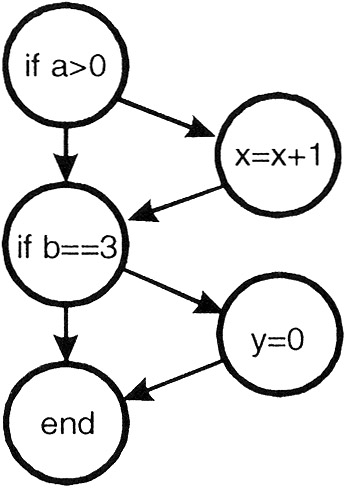Figure 10-2: Graphical representation of the two-line code snippet.

These two lines of code implement four different paths of execution:Figure 10-3: Four execution paths.

While a single test case is sufficient to test every line of code in this module (for example, use a=6 and b=3 as input), it is apparent that this level of coverage will miss testing many paths. Thus, statement coverage, while a beginning, is generally not an acceptable level of testing.

Even though statement coverage is the lowest level of coverage, even that may be difficult to achieve in practice. Often modules have code that is executed only in exceptional circumstances—low memory, full disk, unreadable files, lost connections, etc. Testers may find it difficult or even impossible to simulate these circumstances and thus code that deals with these problems will remain untested.

Holodeck is a tool that can simulate many of these exceptional situations. According to Holodeck's specification it "will allow you, the tester, to test software by observing the system calls that it makes and create test cases that you may use during software execution to modify the behavior of the application. Modifications might include manipulating the parameters sent to functions or changing the return values of functions within your software. In addition, you may also set error-codes and other system events. This set of possibilities allows you to emulate environments that your software might encounter - hence the name 'Holodeck.' Instead of needing to unplug your network connection, create a disk with bad sectors, corrupt packets on the network, or perform any outside or special manipulation of your machine, you can use Holodeck to emulate these problems. Faults can easily be placed into any software testing project that you are using with Holodeck."

Holodeck

Level 0

• Actually, there is a level of coverage below "100% statement coverage." That level is defined as "test whatever you test; let the users test the rest." The corporate landscape is strewn with the sun-bleached bones of organizations who have used this testing approach. Regarding this level of coverage, Boris Beizer wrote "testing less than this [100% statement coverage] for new software is unconscionable and should be criminalized. ... In case I haven't made myself clear, ... untested code in a system is stupid, shortsighted, and irresponsible."

Level 2

• The next level of control flow coverage is "100% decision coverage." This is also called "branch coverage." At this level enough test cases are written so that each decision that has a TRUE and FALSE outcome is evaluated at least once. In the previous example this can be achieved with two test cases (a=2, b=2 and a=4, b=3).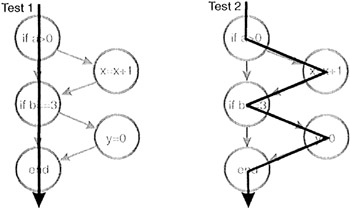Figure 10-4: Two test cases that yield 100% decision coverage.

Case statements with multiple exits would have tests for each exit. Note that decision coverage does not necessarily guarantee path coverage but it does guarantee statement coverage.

Level 3

• Not all conditional statements are as simple as the ones previously shown. Consider these more complicated statements:

``` if (a>0 && c==1) {x=x+1;}
if (b==3 || d<0) {y=0;}
```

To be TRUE, the first statement requires a greater than 0 and c equal 1. The second requires b equal 3 or d less than 0.

In the first statement if the value of a were set to 0 for testing purposes then the c==1 part of the condition would not be tested. (In most programming languages the second expression would not even be evaluated.) The next level of control flow coverage is "100% condition coverage." At this level enough test cases are written so that each condition that has a TRUE and FALSE outcome that makes up a decision is evaluated at least once. This level of coverage can be achieved with two test cases (a>0, c=1, b=3, d<0 and a≤0, c≠1, b≠3, d≥0). Condition coverage is usually better than decision coverage because every individual condition is tested at least once while decision coverage can be achieved without testing every condition.

Level 4

• Consider this situation:

``` if(x&&y) {conditionedStatement;}
// note: && indicates logical AND
```

We can achieve condition coverage with two test cases (x=TRUE, y=FALSE and x=FALSE, y=TRUE) but note that with these choices of data values the conditionedStatement will never be executed. Given the possible combination of conditions such as these, to be more complete "100% decision/condition" coverage can be selected. At this level test cases are created for every condition and every decision.

Level 5

• To be even more thorough, consider how the programming language compiler actually evaluates the multiple conditions in a decision. Use that knowledge to create test cases yielding "100% multiple condition coverage."

``` if (a>0 && c==1) {x=x+1;}
if (b==3 || d<0) {y=0;}
// note: || means logical OR
```

will be evaluated as: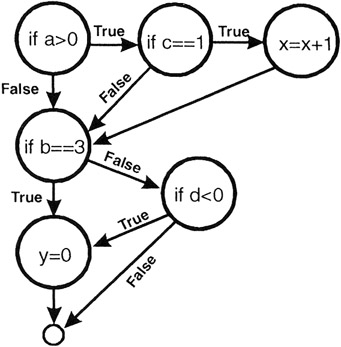Figure 10-5: Compiler evaluation of complex conditions.

This level of coverage can be achieved with four test cases:

``` a>0, c=1, b=3, d<0
a≤0, c=1, b=3, d≥0
a>0, c≠1, b≠3, d<0
a≤0, c≠1, b≠3, d≥0
```

Achieving 100% multiple condition coverage also achieves decision coverage, condition coverage, and decision/condition coverage. Note that multiple condition coverage does not guarantee path coverage.

Level 7

• Finally we reach the highest level, which is "100% path coverage." For code modules without loops the number of paths is generally small enough that a test case can actually be constructed for each path. For modules with loops, the number of paths can be enormous and thus pose an intractable testing problem.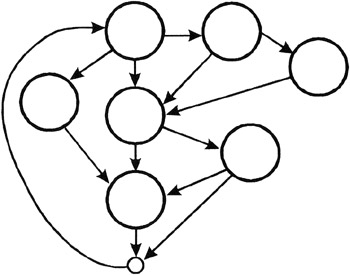Figure 10-6: An interesting flow diagram with many, many paths.

Level 6

• When a module has loops in the code paths such that the number of paths is infinite, a significant but meaningful reduction can be made by limiting loop execution to a small number of cases. The first case is to execute the loop zero times; the second is to execute the loop one time, the third is to execute the loop n times where n is a small number representing a typical loop value; the fourth is to execute the loop its maximum number of times m. In addition you might try m-1 and m+1.

Before beginning control flow testing, an appropriate level of coverage should be chosen.

### Structured Testing Basis Path Testing

No discussion on control flow testing would be complete without a presentation of structured testing, also known as basis path testing. Structured testing is based on the pioneering work of Tom McCabe. It uses an analysis of the topology of the control flow graph to identify test cases.

The structured testing process consists of the following steps:

• Derive the control flow graph from the software module.
• Compute the graph's Cyclomatic Complexity (C).
• Select a set of C basis paths.
• Create a test case for each basis path.
• Execute these tests.

Consider the following control flow graph: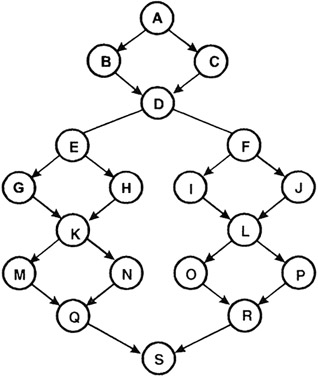Figure 10-7: An example control flow graph.

McCabe defines the Cyclomatic Complexity (C) of a graph as

C = edges - nodes + 2

Edges are the arrows, and nodes are the bubbles on the graph. The preceding graph has 24 edges and 19 nodes for a Cyclomatic Complexity of 24-19+2 = 7.

In some cases this computation can be simplified. If all decisions in the graph are binary (they have exactly two edges flowing out), and there are p binary decisions, then

C = p+1

Cyclomatic Complexity is exactly the minimum number of independent, nonlooping paths (called basis paths) that can, in linear combination, generate all possible paths through the module. In terms of a flow graph, each basis path traverses at least one edge that no other path does.

McCabe's structured testing technique calls for creating C test cases, one for each basis path.

 IMPORTANT ! Creating and executing C test cases, based on the basis paths, guarantees both branch and statement coverage.

Because the set of basis paths covers all the edges and nodes of the control flow graph, satisfying this structured testing criteria automatically guarantees both branch and statement coverage.

A process for creating a set of basis paths is given by McCabe:

1. Pick a "baseline" path. This path should be a reasonably "typical" path of execution rather than an exception processing path. The best choice would be the most important path from the tester's view.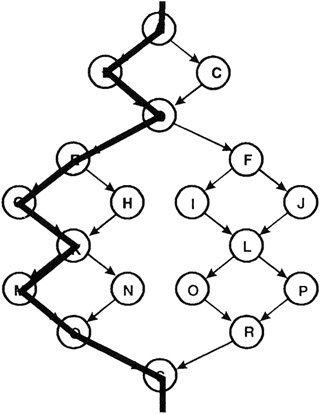Figure 10-8: The chosen baseline basis path ABDEGKMQS

2. To choose the next path, change the outcome of the first decision along the baseline path while keeping the maximum number of other decisions the same as the baseline path.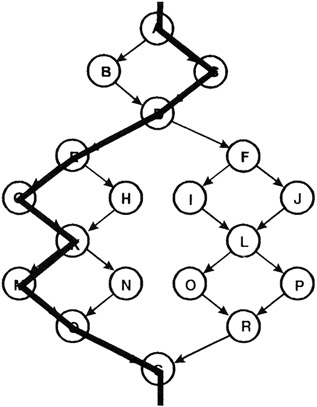Figure 10-9: The second basis path ACDEGKMQS

3. To generate the third path, begin again with the baseline but vary the second decision rather than the first.Figure 10-10: The third basis path ABDFILORS

4. To generate the fourth path, begin again with the baseline but vary the third decision rather than the second. Continue varying each decision, one by one, until the bottom of the graph is reached.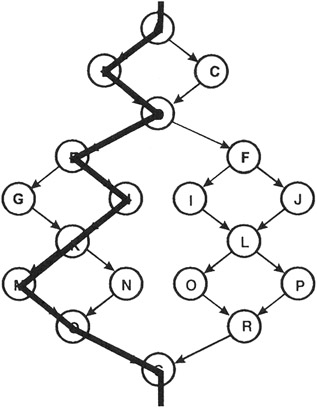Figure 10-11: The fourth basis path ABDEHKMQS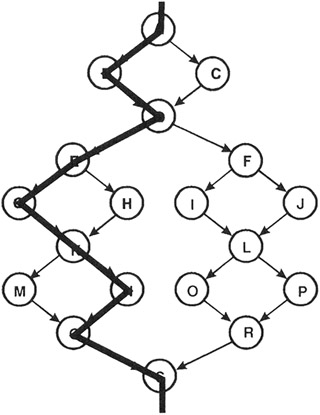Figure 10-12: The fifth basis path ABDEGKNQS

5. Now that all decisions along the baseline path have been flipped, we proceed to the second path, flipping its decisions, one by one. This pattern is continued until the basis path set is complete.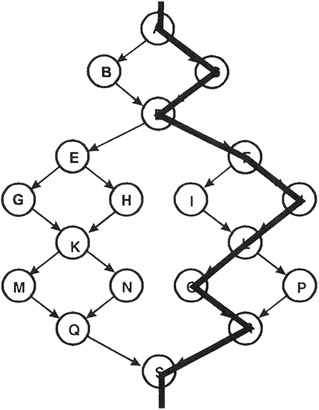Figure 10-13: The sixth basis path ACDFJLORS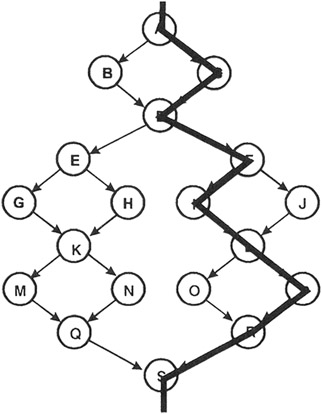Figure 10-14: The seventh basis path ACDFILPRS

Thus, a set of basis paths for this graph are:

• ABDEGKMQS
• ACDEGKMQS
• ABDFILORS
• ABDEHKMQS
• ABDEGKNQS
• ACDFJLORS
• ACDFILPRS

Structured testing calls for the creation of a test case for each of these paths. This set of test cases will guarantee both statement and branch coverage.

Note that multiple sets of basis paths can be created that are not necessarily unique. Each set, however, has the property that a set of test cases based on it will execute every statement and every branch.

## Example

Consider the following example from Brown & Donaldson. It is the code that determines whether B&D should buy or sell a particular stock. Unfortunately, the inner workings are a highly classified trade secret so the actual processing code has been removed and generic statements like s1; s2; etc. have substituted for them. The control flow statements have been left intact but their actual conditions have been removed and generic conditions like c1 and c2 have been put in their place. (You didn't think we'd really show you how to know whether to buy or sell stocks, did you?)

 Note s1, s2, ... represent Java statements while c1, c2, ... represent conditions.
``` boolean evaluateBuySell (TickerSymbol ts) {
s1;
s2;
s3;
if (c1) {s4; s5; s6;}
else {s7; s8;}
while (c2) {
s9;
s10;
switch (c3) {
case-A:
s20;
s21;
s22;
break; // End of Case-A
case-B:
s30;
s31;
if (c4) {
s32;
s33;
s34;
}
else {
s35;
}
break; // End of Case-B
case-C:
s40;
s41;
break; // End of Case-C
case-D:
s50;
break; // End of Case-D
} // End Switch
s60;
s61;
s62;
if (c5) {s70; s71; }
s80;
s81;
} // End While
s90;
s91;
s92;
return result;
```

Figure 10-15: Java code for Brown & Donaldson's evaluateBuySell module.

The following flow diagram corresponds to this Java code: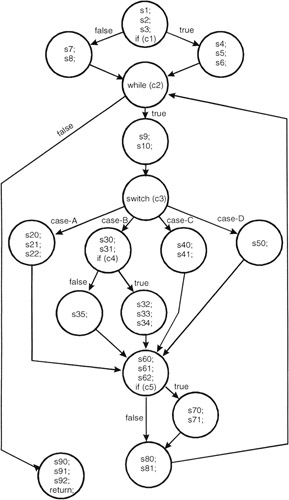Figure 10-16: Control flow graph for Brown & Donaldson's evaluateBuySell module.

The cyclomatic complexity of this diagram is computed by

edges - nodes + 2

or

22-16+2 = 8

Let's remove the code and label each node for simplicity in describing the paths.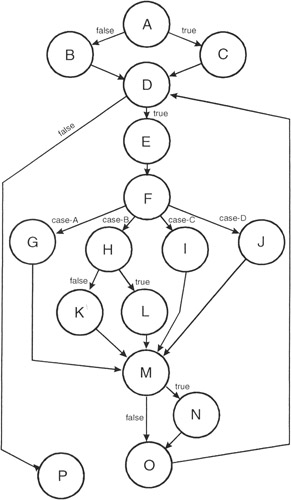Figure 10-17: Control flow graph for Brown & Donaldson's evaluateBuySell module.

A set of eight basis paths is:

1. ABDP
2. ACDP
3. ABDEFGMODP
4. ABDEFHKMODP
5. ABDEFIMODP
6. ABDEFJMODP
7. ABDEFHLMODP
8. ABDEFIMNODP

Remember that basis path sets are not unique; there can be multiple sets of basis paths for a graph.

This basis path set is now implemented as test cases. Choose values for the conditions that would sensitize each path and execute the tests.

Table 10-1: Data values to sensitize the different control flow paths.

Test Case

C1

C2

C3

C4

C5

1

False

False

N/A

N/A

N/A

2

True

False

N/A

N/A

N/A

3

False

True

A

N/A

False

4

False

True

B

False

False

5

False

True

C

N/A

False

6

False

True

D

N/A

False

7

False

True

B

True

False

8

False

True

C

N/A

True

## Applicability and Limitations

Control flow testing is the cornerstone of unit testing. It should be used for all modules of code that cannot be tested sufficiently through reviews and inspections. Its limitations are that the tester must have sufficient programming skill to understand the code and its control flow. In addition, control flow testing can be very time consuming because of all the modules and basis paths that comprise a system.

## Summary

• Control flow testing identifies the execution paths through a module of program code and then creates and executes test cases to cover those paths.
• Control flow graphs are the foundation of control flow testing. Modules of code are converted to graphs, the paths through the graphs are analyzed, and test cases are created from that analysis.
• Cyclomatic Complexity is exactly the minimum number of independent, nonlooping paths (called basis paths) that can, in linear combination, generate all possible paths through the module.
• Because the set of basis paths covers all the edges and nodes of the control flow graph, satisfying this structured testing criteria automatically guarantees both branch and statement coverage.

## Practice

1. Below is a brief program listing. Create the control flow diagram, determine its Cyclomatic Complexity, choose a set of basis paths, and determine the necessary values for the conditions to sensitize each path.
``` if (c1) {
while (c2) {
if (c3) { s1; s2;
if (c5) s5;
else s6;
else
if (c4) { }
else { s3; s4; break;}
} // End of while
} // End of if
s7;
if (c6) s8; s9;
s10;
```

## References

Beizer, Boris (1990). Software Testing Techniques (Second Edition). Van Nostrand Reinhold.

Myers, Glenford (1979). The Art of Software Testing. John Wiley & Sons.

Pressman, Roger S. (1982). Software Engineering: A Practitioner's Approach (Fourth Edition). McGraw-Hill.

Watson, Arthur H. and Thomas J. McCabe. Structured Testing: A Testing Methodology Using the Cyclomatic Complexity Metric. NIST Special Publication 500-235 available at http://www.mccabe.com/nist/nist_pub.php

Preface

Section I - Black Box Testing Techniques

Section II - White Box Testing Techniques

Section IV - Supporting Technologies

Section V - Some Final Thoughts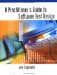A Practitioners Guide to Software Test Design
ISBN: 158053791X
EAN: 2147483647
Year: 2003
Pages: 161
Authors: Lee Copeland